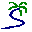# Model entry

Spatial diffsion over a grid: using an array to handle interactions between cells - Model catalogue - Simulistics.com

 Search Simulistics Model catalogue Listed by keyword Listed by ID Listed by title Listed by date added

# Spatial diffsion over a grid: using an array to handle interactions between cells

Simile version : 3.1+
Keywords : Submodel ; Multi-instance submodel ; Spatial modelling ;

## Description

This simulates diffusion over a grid of cells. We use a multiple-instance submodel to represent the set of cells. Each cell has a unique (row,column) attribute. The amount of substance flowing out of each cell is held outside the submodel in an array (with as many elements as there are cells), and this is used to calculate the amount flowing into neighbouring cells.

## Files

### Model file

Click on the icon to download the model file. (You will need Simile to examine and run the model. A free evaluation version is available from the products page.)

Some browsers may attempt to display the model file, rather than open it in Simile; in this case, use the browser back button to return to this page, and use the context menu (invoked by right-clicking on the link) to save the target file to disk.gridspread1.sml

## Diagram## Equations

```Equations in ../Desktop
interflows = [outflow]/8
where: [outflow] = cell/outflow]
rows = [row]
where: [row] = cell/row
cols = [col]
where: [col] = cell/col

Equations in cell
inflow = sum(if abs([rows]-row)<1.5 and abs([cols]-col)<1.5 then [interflows] else 0)
where:
[interflows] = ../interflows
[rows] = ../rows,[cols] = ../cols
outflow = 0.05*amount
row = floor((index(1)-1)/10)+1
col = index(1)-10*(row-1)
compartment:amount = (if index(1) == 35 then 100 else 0)
```